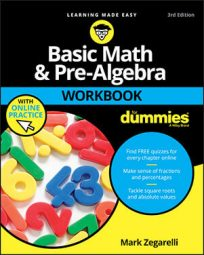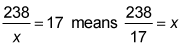##### Basic Math & Pre-Algebra Workbook For Dummies with Online PracticeWhen dealing with simple algebraic expressions, you don't always need algebra to solve them. The following practice questions ask you to use three different methods: inspecting, rewriting the problem, and guessing and checking.

## Practice questions

In the following questions, solve for x in each case just by looking at the equation.

1. 18 – x = 12

2. 4x = 44

In the following questions, use the correct inverse operation to rewrite and solve each problem.

3. 100 – x = 58

4. 238/x = 17

In the following questions, find the value of x in each equation by guessing and checking.

5. 12x – 17 = 151

6. 19x – 8 = 600

## Answers and explanations

1. x = 6

You can solve this problem through simple inspection. Because 18 – 6 = 12, x = 6.

2. x = 11

Again, through simple inspection, because 4(11) = 44, you know that x = 11.

3. x = 42

Turn the problem around by changing the subtraction to addition: 100 – x = 58 means the same thing as 100 – 58 = x, so x = 42.

4. x = 14

Turn the problem around by switching around the division:so x = 14.

5. x = 14

Guess what you think the answer may be. For example, perhaps it's x = 10:

12(10) – 17 = 120 – 17 = 103

103 is less than 151, so this guess is too low. Try x = 20:

12(20) – 17 = 240 – 17 = 223

223 is greater than 151, so this guess is too high. Therefore, x is between 10 and 20. Try x = 15:

12(15) – 17 = 180 – 17 = 163

163 is a little greater than 151, so this guess is a little too high. Try x = 14:

12(14) – 17 = 168 – 17 = 151

151 is correct, so x = 14.

6. x = 32

Again, start by guessing. First, try x = 10:

19(10) – 8 = 190 – 8 = 182

182 is much less than 600, so this guess is much too low. Try x = 30:

19(30) – 8 = 570 – 8 = 562

562 is still less than 600, so this guess is still too low. Try x = 35:

19(35) – 8 = 665 – 8 = 657

657 is greater than 600, so this guess is too high. Therefore, x is between 30 and 35. Try x = 32:

19(32) – 8 = 608 – 8 = 600

600 is correct, so x = 32.